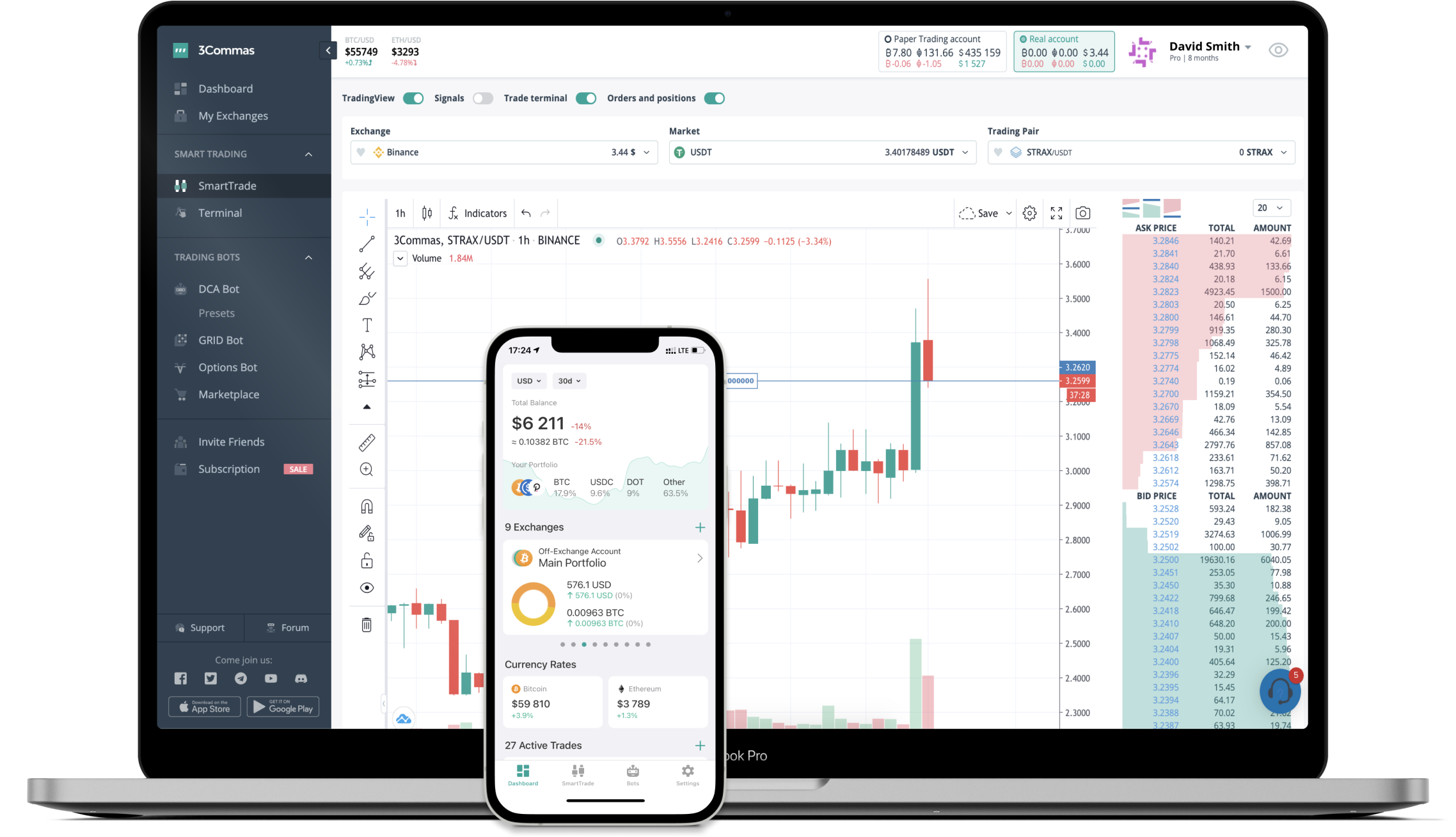# EQUAL to BRL Converter

Equalizer DEX
PriceBRL 38.54-12.17%
VolumeBRL 1,812,256
How it work

The 3Commas currency calculator allows you to convert a currency from Equalizer DEX (EQUAL) to Brazilian Real (BRL) in just a few clicks at live exchange rates.

Simply enter the amount of Equalizer DEX you wish to convert to BRL and the conversion amount automatically populates. You can also use our Prices Calculator Table to calculate how much your currency is worth in other denominations, i.e. .1 EQUAL, .5 EQUAL, 1 EQUAL, 5 EQUAL, or even 10 EQUAL.

## EQUAL to BRL Exchange Calculator

The chart displays Equalizer DEX price changes in BRL.

The ultimate tools for crypto traders to maximize trading profits while minimizing risk and loss.## Latest EQUAL to BRL price calculator

Equalizer DEX market price is updated every three minutes and is automatically displayed in BRL. Below are the most popular denominations to convert to BRL.

Equalizer DEX
Brazilian Real
0.39
BRL
3.85
BRL
38.54
BRL
77.08
BRL
115.62
BRL
192.70
BRL
385.40
BRL
963.50
BRL
1927.00
BRL
3854.00
BRL
9635.00
BRL
19270.00
BRL
38540.00
BRL
96350.00
BRL
Brazilian Real
Equalizer DEX
0.00025947
EQUAL
0.00259471
EQUAL
0.02594707
EQUAL
0.05189414
EQUAL
0.07784120
EQUAL
0.12973534
EQUAL
0.25947068
EQUAL
0.64867670
EQUAL
1.29735340
EQUAL
2.59470680
EQUAL
6.48676700
EQUAL
12.97353399
EQUAL
25.94706798
EQUAL
64.86766995
EQUAL

## Top Currencies